# Astronomy 12 - Spring 1999 (S.T. Myers)

## Solutions to Final Extra Credit Set

Solutions:
1. The volume of the uniform spherical cloud of radius 0.5 pc is

V   =   4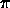R3 / 3   =   1.54 x 1049 m3

which give a mass density for 250 Msun of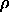=   M / V   =   3.25 x 10-17 kg m-3

which for molecular hydrogen H2 gives a number density

n   =/ mH2   =   ( 3.25 x 10-17 kg m-3 ) / ( 2 x 1.67 x 10-27 kg )   =   9.73 x 109 m-3

and a total of

N   =   n V   =   ( 9.73 x 109 m-3 ) ( 1.54 x 1049 m3 )   =   1.50 x 1059

hydrogen molecules in the cloud. If each molecule has a thermal energy of 1.5 k T, then the total thermal energy K is

K   =   1.5 N k T   =   1.5 x 1.50 x 1059 x 1.38 x 10-23 J K-1 x 10 K   =   3.10 x 1037 J

for the cloud. The gravitational potential energy U is

U   =   -0.6 G M2 / R   =   - 6.48 x 1038 J

so we compare

-U   =   6.48 x 1038 J
vs.
2K   =   6.20 x 1037 J

and since -U > 2K we should have gravitational collapse.

Neglecting internal gas pressure, the globule should collapse on the free-fall timescale (derived from an orbit of a=R/2 and P=2t_ff)

G M ( 2tff )2 = 42 ( R / 2 )3   ==>   ( M / Msun ) ( 2 tff / 1 yr )2 = ( R / 2 AU )3

normalized to the Earth's orbit around the Sun, or

tff   =   ( 1 / 32 )1/2 · ( R / 1 AU )3/2 ( M / Msun )-1/2 yrs   =   1.66 x 107 yrs · ( R / 1 pc )3/2 ( M / Msun )-1/2

which gives

tff = 1.66 x 107 yrs · ( 0.5 )3/2 ( 250 )-1/2 = 3.7 x 105 yrs

and thus the globule should be the site of star formation in less than 1 million years!

2. The circular velocity is

vcirc2 = G M / R

so

M ( < R ) = vcirc2 R / G   ==>   M = Msun ( v / 29.8 km/s )2 ( R / 1 AU )

scaling to the Earth's orbit, or

M   =   9.00 x 1010 Msun · ( v / 222 km/s )2 ( R / 8 kpc )

within the solar circle. The circumference of the solar circle is

2R = 1.55 x 1018 km   ==>   P = ( 1.55 x 1018 km ) / ( 222 km/s ) = 7.05 x 1015 s = 223 x 106 yrs

and thus the Sun makes an orbit of our galaxy every 223 million years. In the current age of the Sun of 4.5 billion years, it has made 20 orbits, and thus our Sun is only 20 G-years old!

You should draw a right triangle with the Sun at the vertex with an angle of 30 degrees between the side Sun-GC and Sun-cloud, and the right angle on the Sun-cloud-GC vertex. With this construction, we see that the distance r of the cloud from the galactic center GC is

r   =   R sin 30°   =   0.5 R   =   4 kpc

for a galactocenter distance R = 8 kpc of the Sun. The projected velocity of the Sun-cloud radial line is equal to the observed velocity of 90 km/s, which is the difference of the projected Sun velocity and the true cloud velocity

v_r   =   v sin 90°   -   220 km/s · sin 30°   =   90 km/s

(since the line of sight is on the spinward side of the GC) or

v   =   220 km/s · 0.5 + 90 km/s   =   200 km/s

for the rotational velocity of the cloud on the 4 kpc circle, which by our above relation means

M ( < r )   =   9.00 x 1010 Msun · ( 200 km/s / 220 km/s )2 ( 4 kpc / 8 kpc )   =   3.72 x 1010 Msun

within the inner 4 kpc of our galaxy.

3. If you start with all hydrogen (1.007825 amu) and fuse 56 hydrogen to 1 iron

 56 1H =   56 x 1.007825 amu   = 56.4382 amu 56Fe = 55.8470 amu ___________________________________________ 0.5912 amu

or a mass fraction of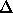m / 56mH   =   0.5912 / 56.4382   =   0.0105

and thus 1.05% of the initial hydrogen mass is turned into energy. Or, more usefully for usm / mFe   =   0.5912 / 55.847   =   0.0106

or 1.06% of the resulting mass of Iron was liberated. Therefore, the formation of 0.02 Msun of iron (2% of 1 Msun) gave off

0.0106 x 0.02 Msun c2   =   3.81 x 1043 J

which corresponds to an average luminosity of

L   ~   ( 3.81 x 1043 J ) / ( 3.156 x 107 s/yr x 108 yr )   =   1.21 x 1028 W   =   31.5 Lsun

over the estimated lifetime of 10^8 years. Note that every 1 Msun of iron out there means 1.91 x 1045 J of energy was released, or 158 Lsun Gyr of energy!

4. For a recession (Hubble) velocity of 12000 km/s, the distance is

d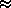v / H0   =   ( 12000 km/s ) / ( 60 km/s/Mpc ) = 200 Mpc

and thus its redshift is

zv / c   =   ( 12000 km/s ) / ( 300000 km/s ) = 0.04

neglecting relativistic and cosmological corrections ( z << 1 ).

Our peculiar velocity of 570 km/s, if it were built up from our Galaxy falling into the potential of the Great Attractor from infinite distance, would be equal to the escape velocity at this distance:

vpec2 ~ vesc2 = 2 G M / R   ==>   M ~ vpec2 R / 2G

or

M   =   7.5 x 1015 Msun · ( v / 570 km/s )2 ( R / 200 Mpc )

and thus the Great Attractor has the mass of several large clusters like Coma!

5. The luminosity, relative to the Sun (+4.83) is

L / Lsun   =   10(4.83+10)/2.5   =   105.932 = 8.55 x 105

which is in line with assuming that there are about 10^6 stars in a globular cluster. If the cluster were at a redshift of z=0.1, then its distance is given by the luminosity distance of

dL   =   (1+z)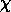=   ( 2 c / H0 ) [ (1+z) - (1+z)1/2 ]

(for a critical universe) which gives

dL   =   ( c / H0 ) · ( 2 [ (1+z) - (1+z)1/2 ] ) = 0.102 c / H0   =   512 Mpc

which is only 0.2% different than the non-cosmological approximation of

d   ~   c z / H0   =   500 km/s

for z = 0.1. The distance modulus should therefore be

mv - Mv   =   5 log( 512 x 106 ) - 5   =   38.5

and so the globular cluster would have an apparent visual magnitude of

mv   =   -10   +   38.5   =   28.5

which is within the magnitude range of HST.

6. We can just go ahead and use our rotation curve - mass relation from Problem 2 again, with new numbers:

M ( < r )   =   9.00 x 1010 Msun · ( 220 km/s / 220 km/s )2 ( 20 kpc / 8 kpc )   =   2.25 x 1011 Msun

within 20 kpc. We then adapt our free-fall time equation from Problem 1 for this mass and a radius of 100 kpc to get

tff   =   1.66 x 107 yrs · ( 100 x 103 pc / 1 pc )3/2 ( 2.25 x 1011 Msun / 1 Msun )-1/2   =   1.11 x 109 yrs

for the estimated formation time for our galaxy. (Note: this problem was particularly easy because we could reuse our scaling relations worked out in previous problems. Whenever you can, derive useful scalings for the quantities from the problem rather than just crunching through it, as you might find something useful in later work.)

Rsw   =   2 G M / c2 = 2.96 km · ( M / Msun )   =   17.8 km · ( M / 6 Msun )

and our black hole has a radius of nearly 18 km. Kepler's law

P   =   1 yr · [ ( a / 1 AU )3 / ( M / Msun ) ]1/2

for an orbit of semimajor axis

a   =   10 Rsw   =   178 km

with a total binary system mass of 12 Msun gives

P   =   3.156 x 107 s · ( 178 km / 1.496 x 108 km )3/2 ( 12 )-1/2   =   0.0118 s

and thus the timescale for gravity wave emissions from a black hole merger is 12 ms, corresponding to a frequency 1/P = 86 Hz!

8. The Wien law gives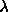max   =   2.89 x 10-3 m / ( T / 1 K ) = 1.059 x 10-3 m

or just over 1 mm wavelength for the peak of the Cosmic Microwave Background emission. At 1 cm, we are in the Rayleigh-Jeans regime and we can easily compute the intensity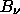2 k T /2   =   7.53 x 10-19 W m-2 Hz-1 sr-1 · ( T / 2.728 K ) (/ 1 cm )-2

or 7.53 MJy/sr for the intensity. Over the whole sky (all 4pi steradians, not just the hemisphere of the sky visible from the surface of Earth) this gives a flux density of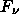=   4=   946 MJy

for the CMB. Note that the Sun is a blackbody with 5770 K and Jupiter with 155 K, so we just need to take the ratio of the solid angles times the temperatures to get the flux densities

Sun:  R = 6.96 x 105 km, d = 1 AU = 1.496 x 108 km
==>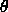= ( 6.96 x 105 / 1.496 x 108 ) rad = 4.65 x 10-3 rad = 960" = 0.27°

which gives the solid angle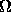=2 = 6.80 x 10-5 sr · ( R / Rsun )2 ( d / 1 AU )-2

and thus for Jupiter ( a = 5.2 AU or d = 4.2 AU from the Earth )

Jup:   R = 71400 km = 0.1026 Rsun, d = 4.2 AU
==>=   6.80 x 10-5 ( 0.1026 )2 ( 4.2 )-2 sr   =   4.06 x 10-8 sr.

The relative flux densities to the all-sky CMB are:

Sun:   Fsun / Fcmb   =   ( 5770 K / 2.728 K ) ( 6.80 x 10-5 sr / 4)   =   0.0114

Jup:   Fjup / Fcmb   =   ( 155 K / 2.728 K ) ( 4.06 x 10-8 sr / 4)   =   1.84 x 10-7 .

Note that the relative surface brightnesses (intensities) are just given by the ratio of temperatures.

The total flux from the sources is just given by the Stefan-Boltzmann law

F = L / ( 4d2 ) =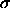T4 ( R / d )2

or

Sun:   F = 1360 W m-2 · ( R / Rsun )2 ( d / 1 AU )-2

and so

Jup:   F = 1360 ( 0.1026 )2 ( 4.2 )-2 W m-2   =   0.812 W m-2

while the CMB effectively deposits its 3 K worth of radiation evenly over the Earth's entire surface and thus

CMB:   F =T4   =   3.14 x 10-6 W m-2

or 3 µW per square meter. (Note that over the entire surface of the Earth, the CMB deposits a total of 1.6 x 10^9 W, or 1.6 GW!)

9. If we rearrange, the equation becomes

dR / R   =   [ 8G u / 3c2 ]1/2 dt

where u depends on which case we are dealing with.

Case 1. Matter Domination: In this case we have

u   =c2   =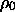( R0 / R )3

which after moving the factors of R over to the left-hand side, gives us the equation to solve

R1/2 dR   =   [ 8G/ 3 ]1/2 R03/2 dt   =   H0 R03/2 dt

since for a critical universe we have the identity

H2 - ( 8G / 3 )= 0

from the energy equation. In any event, we integrate both sides from (t,R) to the current time (t_0,R_0), since we are talking about the late matter-dominated stages, to get

(2/3) [ R03/2 - R3/2 ]   =   H0 [ t0 - t ]

and thus we get the expression

t0 - t   =   (2/3) H0-1 [ 1 - (R/R0)3/2 ]   =   (2/3) H0-1 [ 1 - (1+z)-3/2 ]

for the look-back time. The time scale depends on the Hubble constant as

t0 = (2/3) H0-1   =   2.06 x 1017 h-1 s   =   6.52 x 109 h-1 yrs

if we take t=0 at R=0 under this model (neglecting that eventually the universe becomes radiation dominated).

If recombination occured at a temperature of 3600 K, with a current temperature of 2.73 K, then this must have occured at a scale factor

R0/R = ( 1 + z ) = T/T0   =   ( 3600 / 2.73 ) = 1319

at a redshift z ~ 1318, which puts it at a lookback time of

t0 - t   =   t0 [ 1 - 1319-3/2 ]   =   t0 [ 1 - ( 2.09 x 10-5 ) ]

or a time after the big-bang of

t   =   2.09 x 10-5 t0   =   136000 h-1 yrs

or 227000 years for h=0.6.

Case 2. Radiation Domination: In this instance we clock versus the temperature directly

u   =   a T4   =   a T04 ( R0/R )4

which gives

R dR   =   [ 8G a T04 / 3c2 ]1/2 R02 dt   =   1.61 x 10-20 s-1 · R02 dt

for T_0 = 2.728 K, which we solve by integrating from (t=0,R=0) to (t,R) to get

(1/2) R2   =   1.61 x 10-20 s-1 · R02 t

or

t   =   3.10 x 1019 s · ( R / R0 )2   =   3.10 x 1019 s · ( 1 + z )-2

for the time after the Big Bang.

The Higgs has a mass of 500 GeV / c^2 or an energy of

( 500 x 109 eV ) ( 1.602 x 10-19 J/eV )   =   8.01 x 10-8 J

which corresponds to a thermal energy

k T = 8.01 x 10-8 J   ==>   T = 5.8 x 1015 K

which gives the temperature. The scale factor is

( 1 + z )z   =   ( 5.80 x 1015 K / 2.728 K )   =   2.13 x 1015

or a time of

t   =   ( 3.10 x 1019 s ) ( 2.13 x 1015 )-2   =   6.85 x 10-12 s

for the universe to have a temperature of the Higgs scale.

10. We solve

m   =   h / (c )   =   h / (Rs c )
=   h c2 / ( 2G m c )
==>   m2   =   ( h c ) / ( 2G )

which gives the Planck mass

mpl   =   [ ( h c ) / ( 2G ) ]1/2   =   2.18 x 10-8 kg

which is 10^19 times the proton mass! The corresponding energy scale is

mpl c2   =   1.96 x 109 J   =   1.22 x 1028 eV

which is reached at a temperature of

T   =   mpl c2 / k   =   1.42 x 1032 K

at redshift

( 1 + z )z   =   5.21 x 1031

at a time

t   =   ( 3.10 x 1019 s ) ( 5.21 x 1031 )-2   =   1.14 x 10-44 s

for the universe to have a temperature of the Planck scale.

smyers@nrao.edu   Steven T. Myers